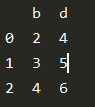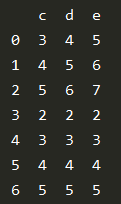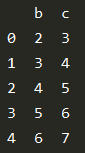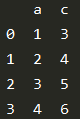# How to take column-slices of DataFrame in Pandas?

• Last Updated : 15 Mar, 2021

In this article, we will learn how to slice a DataFrame column-wise in Python. DataFrame is a two-dimensional tabular data structure with labeled axes .i.e. rows and columns. Slicing of a DataFrame can be done using the following two methods:

### Method #1

Using loc, the loc is present in the pandas package loc can be used to slice a dataframe using indexing. Pandas DataFrame.loc attribute access a group of rows and columns by label(s) or a boolean array in the given DataFrame.

Syntax:

[ : , first : last : step]

Approach:

• Here first indicates the name of the first column to be taken.
• Last indicates the name of the last column to be taken.
• The step indicates the number of indices to advance after each extraction.

Example 1:

## Python3

 `# importing pandas``import` `pandas as pd`` ` `# Using DataFrame() method from pandas module``df1 ``=` `pd.DataFrame({``"a"``: [``1``, ``2``, ``3``], ``"b"``: [``2``, ``3``, ``4``], ``                    ``"c"``: [``3``, ``4``, ``5``], ``"d"``: [``4``, ``5``, ``6``], ``                    ``"e"``: [``5``, ``6``, ``7``]})`` ` `df2 ``=` `df1.loc[:, ``"b"``:``"d"``:``2``]``print``(df2)`

Output:Example 2:

## Python3

 `# importing pandas``import` `pandas as pd`` ` `# Using DataFrame() method from pandas module``df1 ``=` `pd.DataFrame({``"a"``: [``1``, ``2``, ``3``, ``4``, ``5``, ``6``, ``7``], ``                    ``"b"``: [``2``, ``3``, ``4``, ``2``, ``3``, ``4``, ``5``], ``                    ``"c"``: [``3``, ``4``, ``5``, ``2``, ``3``, ``4``, ``5``], ``                    ``"d"``: [``4``, ``5``, ``6``, ``2``, ``3``, ``4``, ``5``], ``                    ``"e"``: [``5``, ``6``, ``7``, ``2``, ``3``, ``4``, ``5``]})`` ` `df2 ``=` `df1.loc[:, ``"c"``:``"e"``:``1``]``print``(df2)`

Output:### Method #2

Using iloc, the iloc is present in the pandas package. The iloc can be used to slice a dataframe using indexing. Dataframe.iloc[] method is used when the index label of a data frame is something other than numeric series of 0, 1, 2, 3….n or in case the user doesn’t know the index label. Rows can be extracted using an imaginary index position that isn’t visible in the data frame.

Syntax:

[ start : stop : step]

Approach:

• Here Start indicates the index of the first column to be taken.
• Stop indicates the index of the last column to be taken.
• The step indicates the number of indices to advance after each extraction.

Example 1:

## Python3

 `# importing pandas``import` `pandas as pd``# Using DataFrame() method from pandas module``df1 ``=` `pd.DataFrame({``"a"``: [``1``, ``2``, ``3``, ``4``, ``5``], ``                    ``"b"``: [``2``, ``3``, ``4``, ``5``, ``6``], ``                    ``"c"``: [``3``, ``4``, ``5``, ``6``, ``7``], ``                    ``"d"``: [``4``, ``5``, ``7``, ``8``, ``9``]})`` ` `df2 ``=` `df1.iloc[:, ``1``:``3``:``1``]``print``(df2)`

Output:Example 2:

## Python3

 `# importing pandas``import` `pandas as pd`` ` `# Using DataFrame() method from pandas module``df1 ``=` `pd.DataFrame({``"a"``: [``1``, ``2``, ``3``, ``4``, ``5``], ``                    ``"b"``: [``2``, ``3``, ``4``, ``5``, ``6``], ``                    ``"c"``: [``3``, ``4``, ``5``, ``6``, ``7``], ``                    ``"d"``: [``4``, ``5``, ``7``, ``8``, ``9``]})`` ` `df2 ``=` `df1.iloc[:, ``0``:``3``:``2``]``print``(df2)`

Output:My Personal Notes arrow_drop_up